top of page

## Interval Identification | Interval Of A Sixth (6 of 10)

Similar to an interval of a second and an interval of a third, an interval of a sixth can also be either Major or minor.

## Minor Sixth Intervals

An interval of a minor sixth occurs when two notes are separated by the distance of eight consecutive half steps.

Remembering that two half steps equals one whole step, eight half steps can also be looked at as being comparatively the same as three whole steps and two half steps.

Using this pattern of steps, let's figure out what an interval of a minor sixth above the note A is.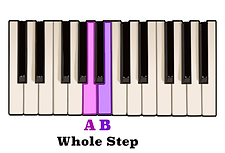One whole step above the note A is the note B.Another whole step above the note D is the note E.A half step above B is the note C.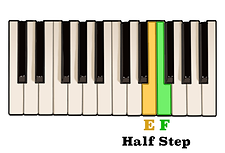And finally, a half step above E is the note F.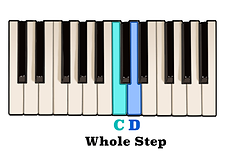The notes C and D are separated by a whole step.As you can see, an interval of a minor sixth above the note A is the note F.

## Major Sixth Intervals

On the other hand, an interval of a Major sixth occurs when two notes are separated by the distance of nine consecutive half steps, or four whole steps and one half step.

Knowing this, let's figure out what an interval of a Major sixth above the note G is.A whole step above the note G is the note A.A whole step above the note C is D.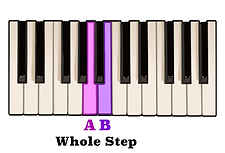Another whole step above A is the note B.And finally, a whole step above the note D is the note E.A half step separates the notes B and C.An interval of a Major sixth above the note G is the note E.

## Relation To The Scale | Minor Sixth Intervals

For certain intervals which are separated by a large amount of steps, such as minor and Major sixths, rather than having to count eight consecutive half steps, using the scale as we had for the previous intervals might be a more practical solution.

For example, we recently learned that an interval of a minor sixth above the note A is the note F. If we take a look at an A minor scale, we can see that the sixth scale degree, or the sixth note in the scale, is in fact the note F.Likewise, if we want to know what an interval of a minor sixth above the note B is, we could simply take a look at a B minor scale and easily see that the sixth scale degree is the note G.## Relation To The Scale | Major Sixth Intervals

This same method may be applied to an interval of a Major sixth as well.

We already know that an interval of a Major sixth above the note G is the note E. Looking at a G Major scale, we can see that the sixth note in the scale is in fact the note E.In the same respect, by looking at the notes of a C Major scale, because A is the sixth note in the scale, we know that an interval of a Major sixth above the note C is the note A.An interval of a sixth is any interval which spans six positions on the staff.

When reading either an interval of a minor or Major sixth on the staff, you will notice that each note will always go from either a line to space, with two spaces and two lines between them,or from a space to a line with two lines and two spaces between them.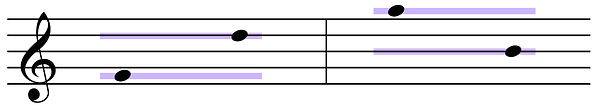As always, it is the performer's job to determine whether the distance between each note is either an interval of a minor sixth, or an interval of a Major sixth. Just like all of the other intervals discussed in the previous videos, it would be very advantageous to memorize this shape so that you will be able to quickly recognize it when reading or writing music.

bottom of page